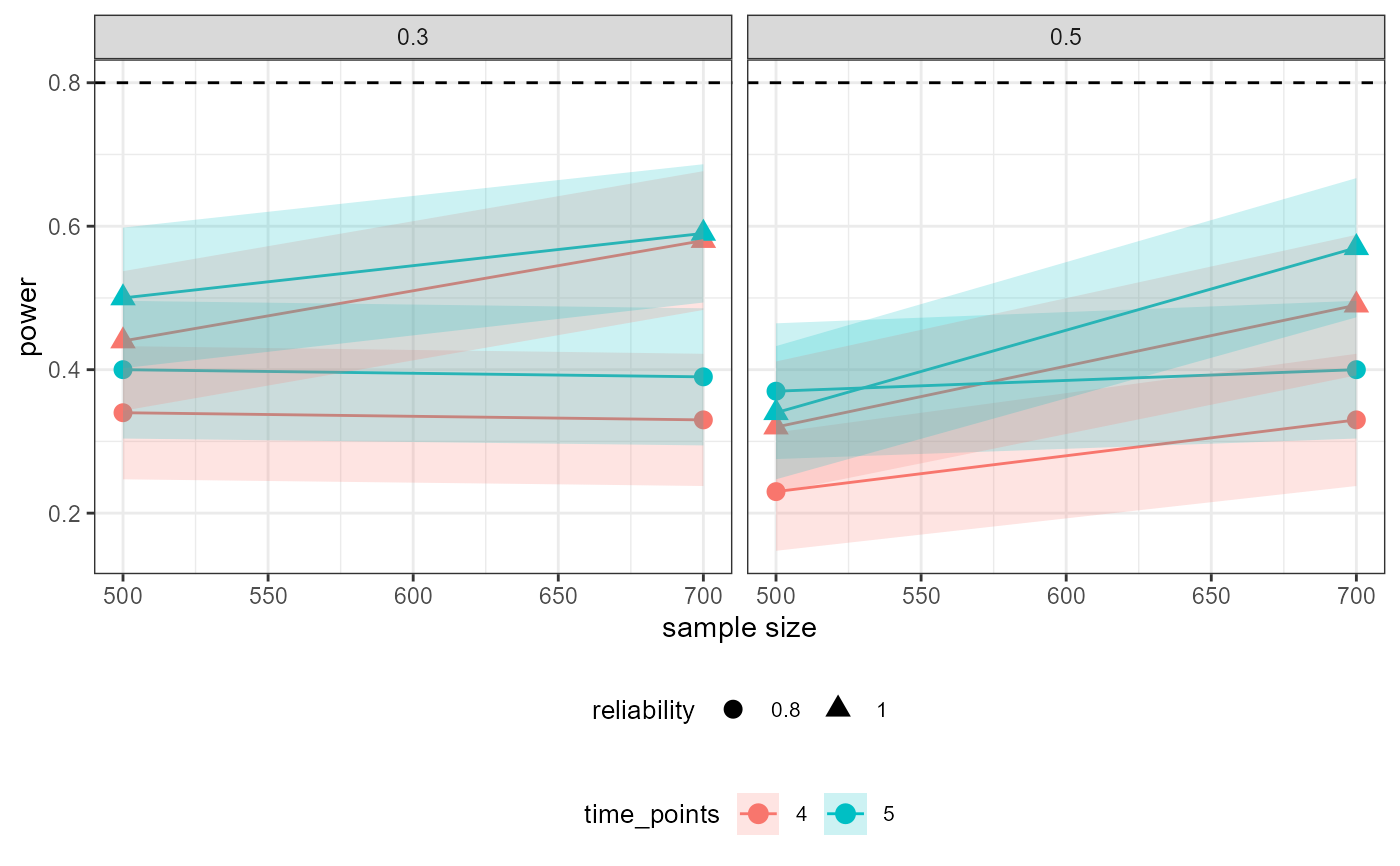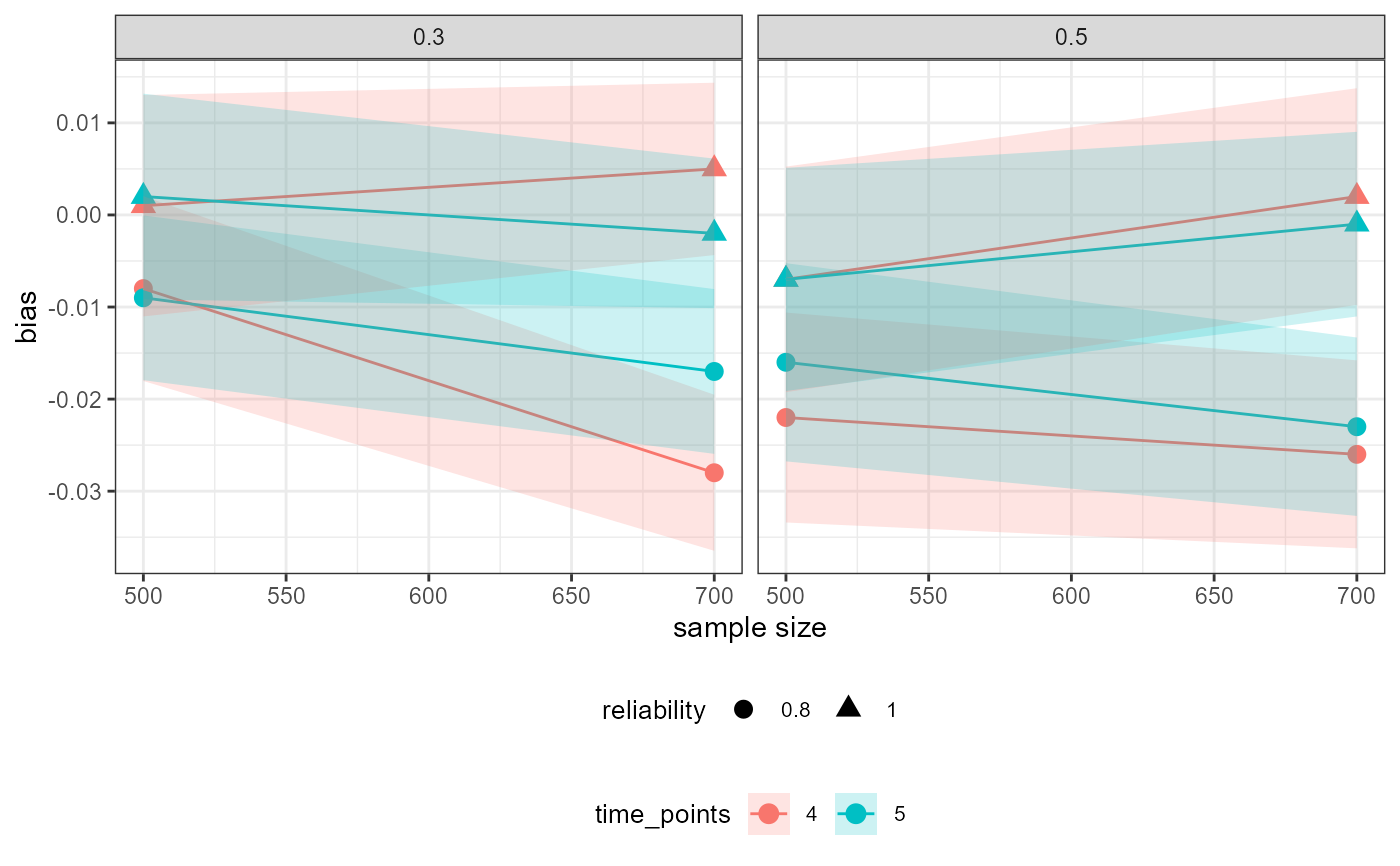Visualizes (using ggplot2) the results from a powRICLPM analysis, for a specific parameter, across all experimental conditions. By default, sample size is plotted on the x-axis, power on the y-axis, and results are grouped by the number of time points and wrapped by the proportion of between-unit variance. Optionally, the y argument can be used to change the variable on the y-axis to other outcomes from the powRICLPM analysis.

## Usage

# S3 method for powRICLPM
plot(x, y = "power", ..., parameter = NULL)

## Arguments

x

A powRICLPM object.

y

(optional) A character string, specifying which outcome is plotted on the y-axis (see "Details").

...

(don't use)

parameter

Character string of length denoting the parameter to visualize the results for.

## Value

A ggplot2 object.

## Details

### y-axis options

The following outcomes can be plotted on the y-axis:

• average: The average estimate.

• MSE: The mean square error.

• coverage: The coverage rate

• accuracy: The average width of the confidence interval.

• SD: Standard deviation of parameter estimates.

• SEAvg: Average standard error.

• bias: The absolute difference between the average estimate and population value.

• give: Extract information (e.g., performance measures) for a specific parameter, across all experimental conditions. This function is used internally in plot.powRICLPM.

## Examples

# \dontshow{
# }
# Visualize power for "wB2~wA1" across simulation conditions
plot(out_preliminary, parameter = "wB2~wA1")# Visualize bias for "wB2~wA1" across simulation conditions
plot(out_preliminary, y = "bias", parameter = "wB2~wA1")# Visualize coverage rate for "wB2~wA1" across simulation conditions
plot(out_preliminary, y = "coverage", parameter = "wB2~wA1")# Visualize MSE for autoregressive effect across simulation conditions
plot(out_preliminary, y = "MSE", parameter = "wA2~wA1")# Error: No parameter specified
try(plot(out_preliminary))
#> Error in check_parameter_given(parameter) : No parameter was specified:
#> ℹ plot() needs to know which specific parameter to create a plot for.